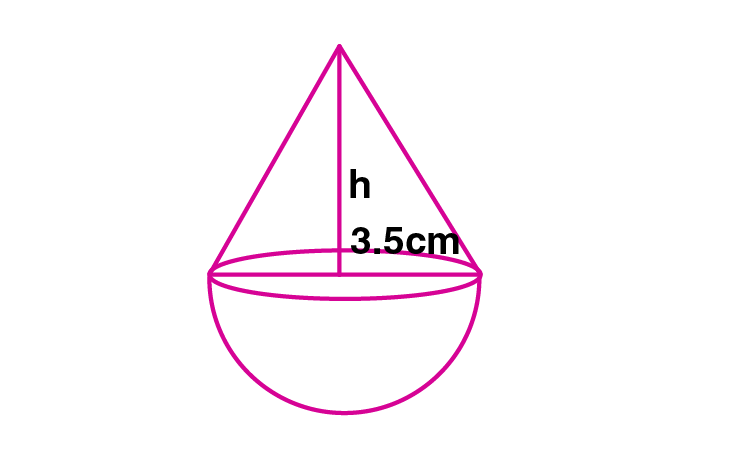Newbie

# A buoy is made in the form of a hemisphere surmounted by a right cone whose circular base coincides with the plane surface of the hemisphere. The radius of the base of the cone is 3.5 metres and its volume is 2/3 of the hemisphere. Calculate the height of the cone and the surface area of the buoy correct to 2 places of decimal.

• 0

This is the Important question of class 10 Based on Mensuration Chapter of M.L Aggarwal book for ICSE BOARD.
Here a buoy is made in the form of a hemisphere surmounted by a right cone whose circular base coincides with the plane surface of the hemisphere. The radius of the base of the cone and its volume is 2/3 of the hemisphere.
This is the Question Number 15, Exercise 17.4 of M.L Aggarwal.

Share

1.Given radius of the cone, r = 3.5 cm

Radius of hemisphere, r = 3.5 cm = 7/2 cm

Volume of hemisphere = (2/3)r3

= (2/3)×(22/7)×(7/2)3

= (2/3)×(22/7)×(7/2)×(7/2)×(7/2)

= (22/3)×(7/2)×(7/2)

= 11×49/6

= 539/6 m3

Volume of cone = 2/3 of volume of hemisphere

= (2/3)× 539/6

= 539/9 m3

Volume of cone = (1/3)r2h

(1/3)r2h = 539/9

(1/3)×(22/7)×(7/2)2×h = 539/9

h = 539×3×2/9×11×7

h = 14/3

h = 4.667

h = 4.67 m

Hence the height of the cone is 4.67 m.

Slant height of cone, l = √(h2+r2)

= √((14/3)2+(7/2)2)

= √((196/9)+(49/4))

= √((784/36)+(441/36))

= √(1225/36)

= 35/6 m

Surface area of the buoy = Surface area of cone + surface area of the hemisphere

= rl + 2r2

= r(l+ 2r)

= (22/7)×(7/2)×((35/6)+2×(7/2))

= 11×((35/6)+7)

= 11×(5.8333+7)

= 11×(12.8333)

= 141.166 m2

= 141.17 m2

Hence the Surface area of the buoy is 141.17 m2.

• 1